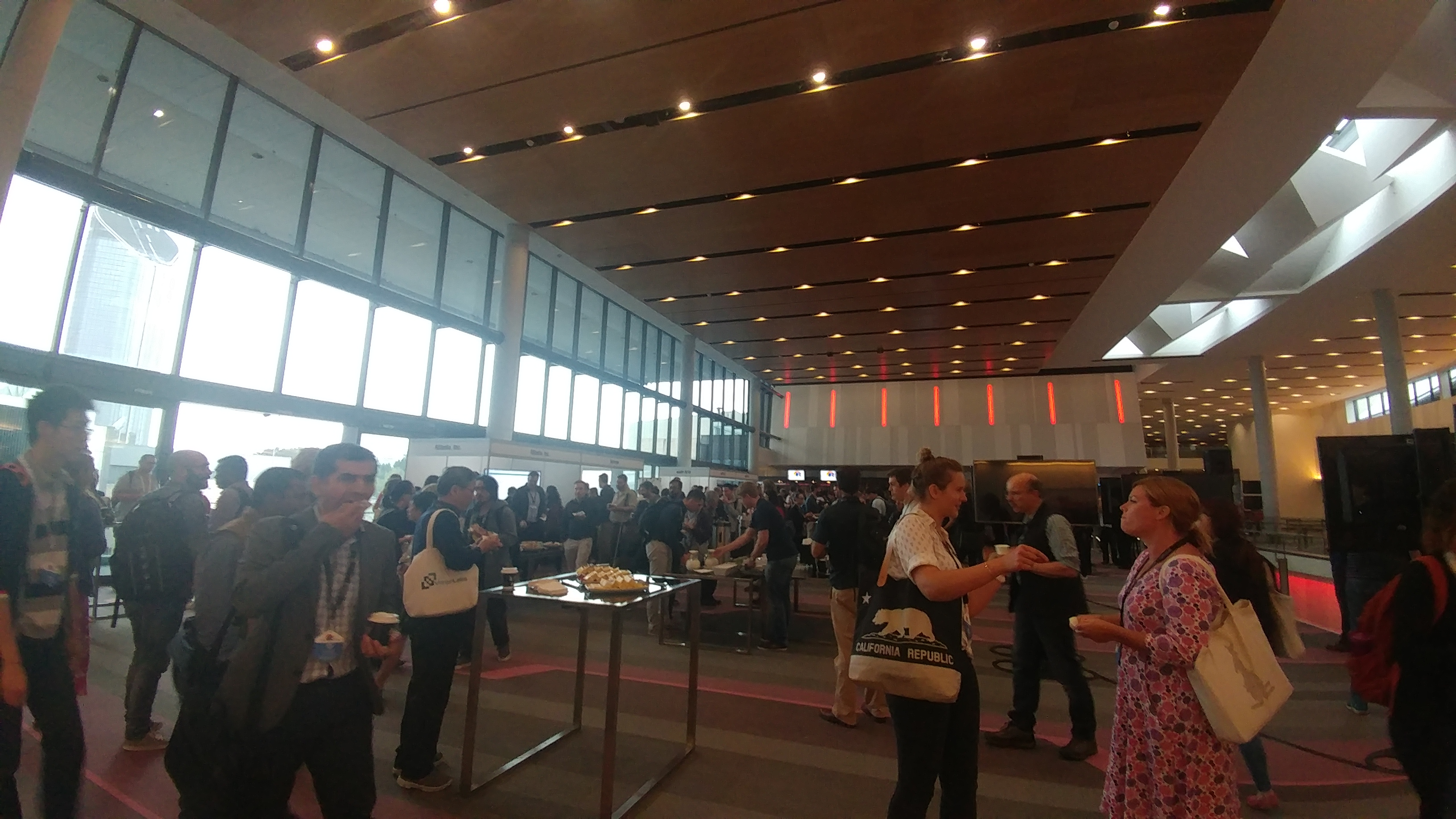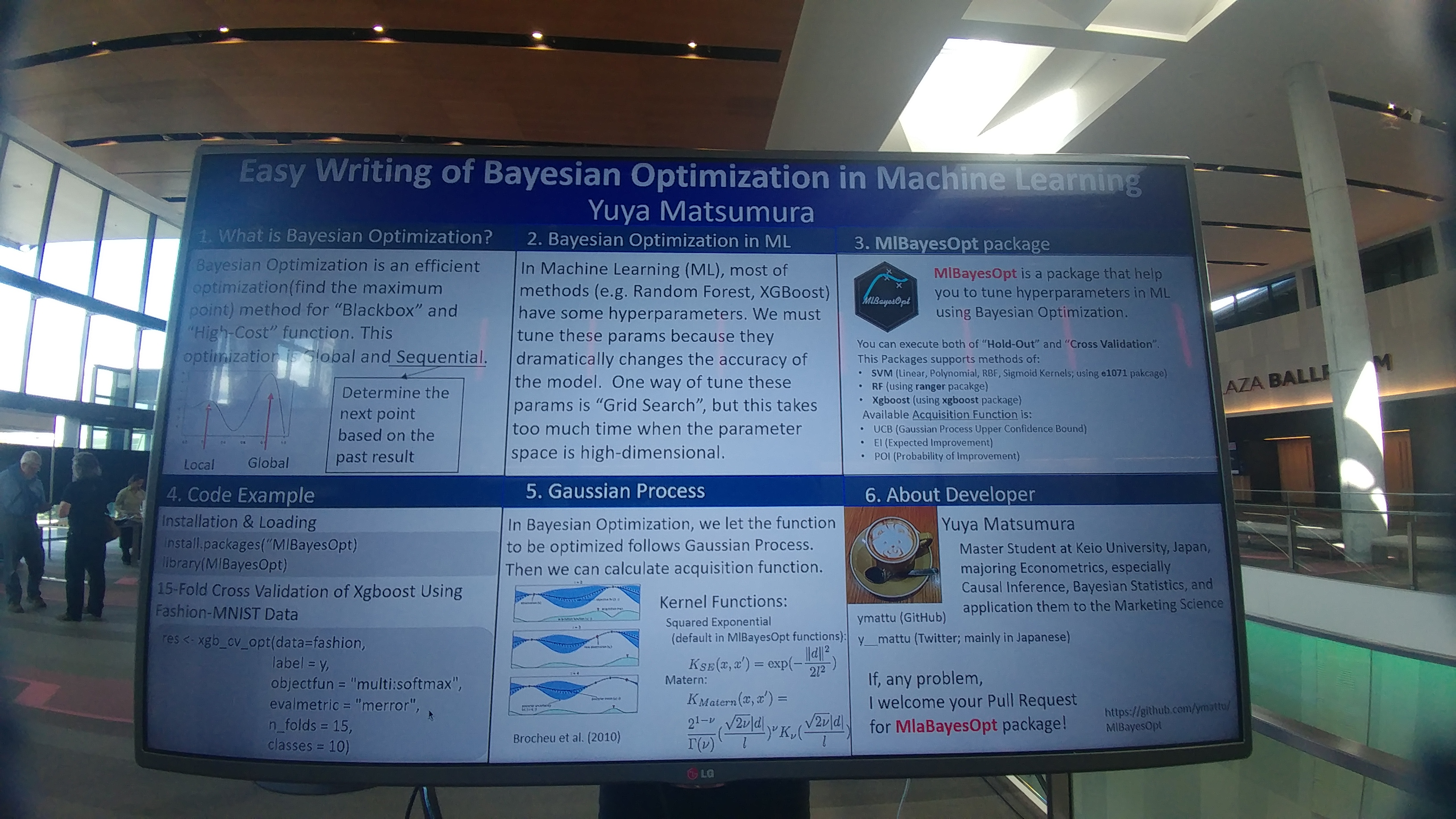# Introduction

## Profile

• Yuya MATSUMURA
(松村優哉)
• GitHub: ymattu
• Graduate student of Keio University
• Studying: Econometrics, Bayesian Statistics, Causal Inference
• Languages: R, Python, SAS# useR!2018

## Fantastic Conference## hexwall## Poster Presentation# Today’s Talk:

## In short…

• Very short summary of poster presentation at useR!2018
• cf. Global TokyoR #2
• Today’s content is updated.

# Summary of this package

• MlBayesOpt(https://github.com/ymattu/MlBayesOpt)
• This package make it easier to write a script to execute parameter tuning using bayesian optimization.
• SVM(Linear, Polynomial, RBF, Sigmoid Kernels)、Random ForestXGboost
• Based on following packages:
• SVM(e1071)
• RF(ranger)
• XGboost(xgboost)
• Bayesian Optimization(rBayesianOptimization)
• Wecan use both of “Hold-Out” and “Cross Validation”

# MlBayesOpt

### Installation

install.packages("MlBayesOpt")
devtools::install_github("ymattu/MlBayesOpt")

library(MlBayesOpt)

# Hold-Out

## SVM

set.seed(123)

res0 <- svm_opt(train_data = iris_train,
train_label = Species,
test_data = iris_test,
test_label = Species,
svm_kernel = "polynomial",
init_points = 10,
n_iter = 1)

## Output of SVM (Excerpt)

elapsed = 0.01  Round = 1   degree_opt = 5.0000 coef0_opt = 1.2641  Value = 0.9333
elapsed = 0.01  Round = 2   degree_opt = 7.0000 coef0_opt = 7.6410  Value = 0.9333
elapsed = 0.01  Round = 3   degree_opt = 5.0000 coef0_opt = 1.5038  Value = 0.9600
elapsed = 0.01  Round = 4   degree_opt = 4.0000 coef0_opt = 7.6924  Value = 0.9333
elapsed = 0.01  Round = 5   degree_opt = 5.0000 coef0_opt = 8.4372  Value = 0.9333
elapsed = 0.01  Round = 6   degree_opt = 10.0000    coef0_opt = 7.7898  Value = 0.9333
elapsed = 0.01  Round = 7   degree_opt = 8.0000 coef0_opt = 1.4249  Value = 0.9200
elapsed = 0.01  Round = 8   degree_opt = 9.0000 coef0_opt = 8.0788  Value = 0.9333
elapsed = 0.01  Round = 9   degree_opt = 5.0000 coef0_opt = 8.9781  Value = 0.9333
elapsed = 0.01  Round = 10  degree_opt = 6.0000 coef0_opt = 9.2771  Value = 0.9333
elapsed = 0.01  Round = 11  degree_opt = 10.0000    coef0_opt = 4.4448  Value = 0.9333

Best Parameters Found:
Round = 3   degree_opt = 5.0000 coef0_opt = 1.5038  Value = 0.9600 

# Cross Validation

## SVM

set.seed(71)
res0 <- svm_cv_opt(data = iris,
label = Species,
n_folds = 3,
init_points = 10,
n_iter = 1)

## Output of SVM

elapsed = 0.02  Round = 1   gamma_opt = 3.3299  cost_opt = 11.7670  Value = 0.9333
elapsed = 0.01  Round = 2   gamma_opt = 5.5515  cost_opt = 76.1740  Value = 0.9067
elapsed = 0.01  Round = 3   gamma_opt = 3.2744  cost_opt = 14.1882  Value = 0.9400
elapsed = 0.01  Round = 4   gamma_opt = 2.1175  cost_opt = 76.6932  Value = 0.9200
elapsed = 0.01  Round = 5   gamma_opt = 3.1619  cost_opt = 84.2154  Value = 0.9600
elapsed = 0.01  Round = 6   gamma_opt = 9.4727  cost_opt = 77.6772  Value = 0.8933
elapsed = 0.01  Round = 7   gamma_opt = 6.6175  cost_opt = 13.3914  Value = 0.9267
elapsed = 0.02  Round = 8   gamma_opt = 8.8943  cost_opt = 80.5955  Value = 0.8733
elapsed = 0.01  Round = 9   gamma_opt = 3.3808  cost_opt = 89.6793  Value = 0.9333
elapsed = 0.01  Round = 10  gamma_opt = 4.3481  cost_opt = 92.6987  Value = 0.9000
elapsed = 0.01  Round = 11  gamma_opt = 2.9508  cost_opt = 84.8600  Value = 0.9467

Best Parameters Found:
Round = 5   gamma_opt = 3.1619  cost_opt = 84.2154  Value = 0.9600 

## XGboost

set.seed(71)

res0 <- xgb_cv_opt(data = iris,
label = Species,
objectfun = "multi:softmax",
evalmetric = "mlogloss",
n_folds = 3,
classes = 3,
init_points = 2,
n_iter = 1)
)

ex. “Hold Out” using SVM

res <- svm_opt(
# about dataset (at least required)
train_data = iris_train,
train_label = Species,
test_data = iris_test,
test_label = Species,
# about hyper parameters (optional, default is following)
svm_kernel = "radial", # RBF
gamma_range = c(10 ^ (-5), 10 ^ 5),
cost_range = c(10 ^ (-2), 10 ^ 2),
# about bayesian optimization (optional, default is following)
init_points = 20,
n_iter = 1,
acq = "ei",
kappa = 2.576,
eps = 0.0,
optkernel = list(type = "exponential", power = 2)
)

# Future Works

## This package is still a development version…

• Fix some bugs
• Make functuions about Deep Learning…? (mxnet package…?)

## Enjoy R programming !

This slide is made from revealjs package.

This slide and Rmd file are published on Git Hub(https://github.com/ymattu/TokyoR71).